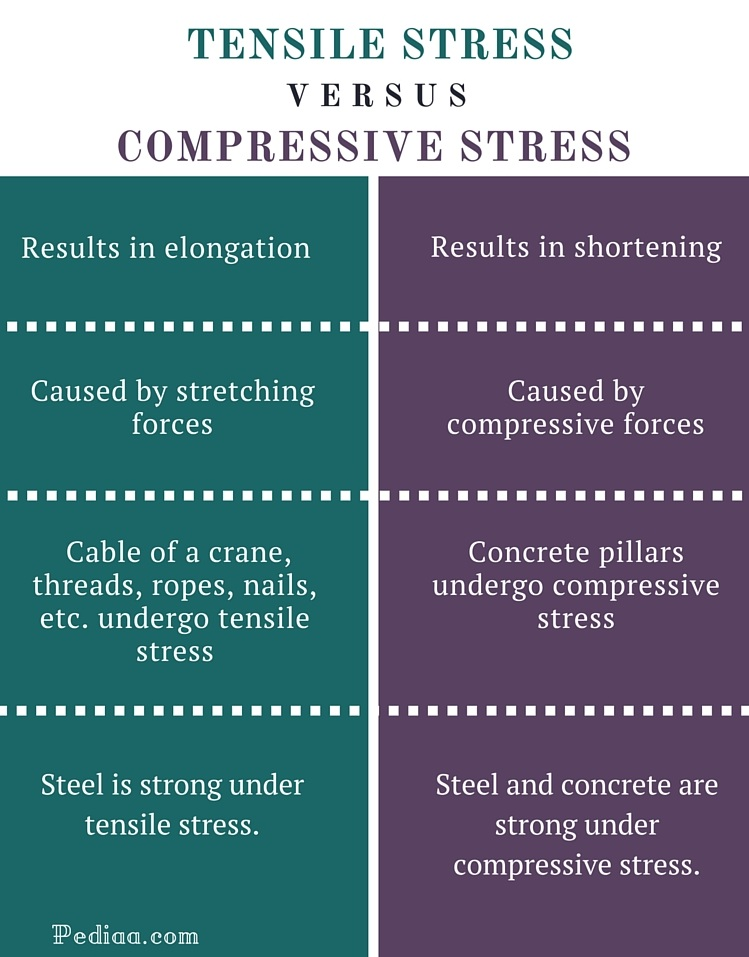# Difference Between Tensile and Compressive Stress

## Main Difference – Tensile vs Compressive Stress

Tensile and compressive stresses are two types of stresses a material can undergo. The type of the stress is determined by the force being applied on the material. If it is a tensile (stretching) force, the material experiences a tensile stress. If it is a compressive (squeezing) force, the material experiences a compressive stress. The main difference between tensile and compressive stress is that tensile stress results in elongation whereas compressive stress results in shortening. Some materials are strong under tensile stresses but weak under compressive stresses. However, materials such as concrete are weak under tensile stresses but strong under compressive stresses. So, these two quantities are very important when choosing suitable materials for applications. The importance of the quantity depends on the application. Some applications require materials that are strong under tensile stresses. But some applications require materials that are strong under compressive stresses, especially in structural engineering.

## What is Tensile Stress

Tensile stress is a quantity associated with stretching or tensile forces. Usually, tensile stress is defined as the force per unit area and denoted by the symbol σ. The tensile stress (σ) that develops when an external stretching force (F) is applied on an object is given by σ = F/A  where A is the cross sectional area of the object. Therefore, the SI unit of measuring tensile stress is Nm-2 or Pa. Higher the load or tensile force, higher the tensile stress. The tensile stress corresponding to the force applied on an object is inversely proportional to the cross sectional area of the object. An object is elongated when a stretching force is applied on the object.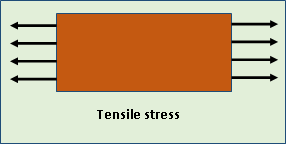The shape of the graph of tensile stress vs. strain depends on the material. There are three important stages of the tensile stress namely yield strength, ultimate strength and the breaking strength (rupture point). These values can be found by plotting the graph of tensile stress vs. strain. The data required to plot the graph is obtained performing a tensile test. The plot of the graph of tensile stress vs. strain is linear up to a certain value of tensile stress, and thereafter it deviates. The Hook’s law is valid only up to that value.

A material which is under a tensile stress returns to its original shape when the load or tensile stress is removed. This ability of a material is known as the elasticity of the material. But the elastic property of a material can be seen only up to a certain value of the tensile stress, called the yield strength of the material. The material losses its elasticity at the point of yield strength. Thereafter, the material undergoes a permanent deformation and does not return to its original shape even if the external tensile force is completely removed. Ductile materials such as gold undergo a notable amount of plastic deformation. But brittle materials such as ceramics undergo a small amount of plastic deformation.

The ultimate tensile strength of a material is the maximum tensile stress that the material can withstand. It is a very important quantity, especially in manufacturing and engineering applications. The breaking strength of a material is the tensile stress at the point of fracture. In some cases, the ultimate tensile stress is equal to the breaking stress.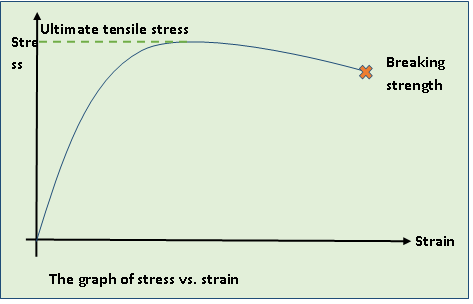## What is Compressive Stress

Compressive stress is the opposite of tensile stress. An object experiences a compressive stress when a squeezing force is applied on the object. So, an object subjected to a compressive stress is shortened. Compressive stress is also defined as the force per unit area and denoted by the symbol σ. The compressive stress (σ) that develops when an external compressive or squeezing force (F) is applied on an object is given by σ = F/A. Higher the compressive force, higher the compressive stress.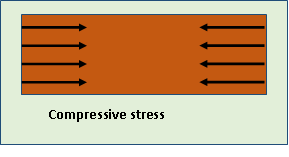The ability of a material to withstand a higher compressive stress is a very important mechanical property, especially in engineering applications. Some materials such as steel are strong under both tensile and compressive stresses. However, some materials such as concrete are strong only under compressive stresses. Concrete is relatively weak under tensile stresses.

When a structural component is bent, it undergoes both lengthening and shortening at the same time. The following figure shows a concrete beam subjected to a bending force. Its upper part is elongated due to the tensile stress whereas the bottom part is shortened due to the compressive stress. Therefore, it is very important to choose a suitable material when designing such structural components. A typical material should be sufficiently strong under both tensile and compressive stresses.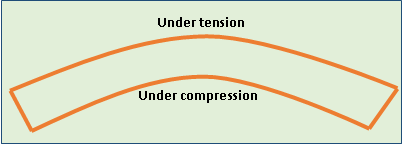## Difference Between Tensile and Compressive Stress

### Physical result:

Tensile stress: Tensile stress results in elongation.

Compressive stress: Compressive stress results in shortening.

### Caused by:

Tensile stress: Tensile stress is caused by stretching forces.

Compressive stress: Compressive stress is caused by compressive forces.

### Objects under stresses:

Tensile stress: Cable of a crane, threads, ropes, nails, etc. undergo tensile stress.

Compressive stress: Concrete pillars undergo compressive stress.

### Strong materials

Tensile stress: Steel is strong under tensile stress.

Compressive stress: Steel and concrete are strong under compressive stress.